“Algorithmic trading is a process for executing orders utilizing automated and pre-programmed trading instructions to account for variables such as price, timing and volume. An algorithm is a set of directions for solving a problem. Computer algorithms send small portions of the full order to the market over time.” - Investopedia.

## Вasic concepts¶

The decision to buy or sell financial securities uses a predetermined algorithm, with the intent to make a profit. On our platform, this algo is a python script which takes historical data as an input and gives a decision to buy/sell as output.

Let us say that we have a capital of 1M USD and we want to invest in a portfolio consisting of three stocks: Apple Inc (AAPL), Alphabet Inc Class C (GOOG), Tesla Inc (TSLA). Let us have a look at the open price of these stocks for some period. The open price is the price at which a security first trades upon the opening of an exchange on a trading day. We use historical data from the NASDAQ exchange as input:

date AAPL GOOG TSLA
Mar 02, 2020 282.28 1,351.61 711.26
Mar 03, 2020 303.67 1,399.42 805.00
Mar 04, 2020 296.44 1,359.23 763.96

Table 1: Stock open price in USD. NASDAQ exchange.

Now we need to define an algorithm which allocates our capital to stocks. The algorithm can be seen as an intelligent search of some world event reflected in data. Suppose that we have a simple hypothesis: invest more if open price is low. This hypothesis can be expressed through the formula:

$\label{alpha1} \frac{1}{\textbf{open}}.$

Hereinafter we will use bold symbols for vectors. We can receive a matrix form of the algorithm by applying formula (2) to the table 1:

date AAPL GOOG TSLA
Mar 02, 2020 0.00354258183 0.00073985839 0.00140595562
Mar 03, 2020 0.00329304837 0.00071458175 0.00124223602
Mar 04, 2020 0.00337336391 0.00073571065 0.00130896905

Table 2: Matrix form of the algorithm.

The capital is distributed in proportion to the values of the matrix. To receive the shares of capital invested in each stock, the matrix (table 2) must be normalized ($$ l_1 $$ norm) to one for each day:

date AAPL GOOG TSLA
Mar 02, 2020 0.6228 0.1301 0.2472
Mar 03, 2020 0.6273 0.1361 0.2366
Mar 04, 2020 0.6226 0.1358 0.2416

Table 3: Final weights of the algorithm.

Thus, $1M·0.6228 = 622,800$ USD were allocated for AAPL on Mar 02, 2020; GOOG and TSLA received $130,100$ USD and $247,200$ USD. The matrix values (3) are called weights of the algorithm.

## Long Position vs. Short Position¶

To sell a stock, one needs simply to assign it a negative weight in the algorithm. Let us assume that you have the following weights:

date AAPL GOOG TSLA
Mar 02, 2020 0.4 0.4 -0.2

The positive sign means that we hold a long position (buy shares); the negative sign means that we hold a short position (sell shares). Thus, the 1M USD capital will be allocated in the following proportions: $400,000$ USD to AAPL; $400,000$ USD to GOOG; $-200,000$ to TSLA.

Details

For our platform, we use a simplified treatment of short positions and mirror long positions. For a real-environment trading one needs to remember that short positions are associated with higher risks. An investor selling shares that he does not yet own (as a rule, from his broker’s account) is obligated to buy them back after a while. Thus, the investor expects a fall in prices and plays against the market. It leads to several risks:

• Some stocks are hard to borrow. The reason is high demand, a limited number of securities and so on. For a short position for such securities, the broker charges an additional fee.

• The short seller is responsible for paying dividends on shares to the person from whom they were borrowed.

• The potential losses in a short sale can be endless, because theoretically the maximum price of a stock is unlimited. On the other hand, the stock price will not fall below zero, so the maximum profit is limited.

• Even if the estimate is correct, the moment may be inappropriate. It is extremely unprofitable to hold a short position at a rising price for a long time.

## Relative returns¶

The investment portfolio is redistributed according to the algorithm once a day (the exchange has 252 working days a year). Redistribution occurs at the beginning of the day. For our platform, we assume that the purchase of shares occurs at the open price.

Equity grows with the correct prediction of price changes. The daily change in equity is estimated after the end of the trading day and strongly depends on the close price. Close is the price at which a security last trades on a trading day.

Algorithm results, calculated on historical data, are usually presented on an equity graph in order to understand the behaviour of the cumulative profit. In our platform, we set initial equity to 1, so it can be scaled easily.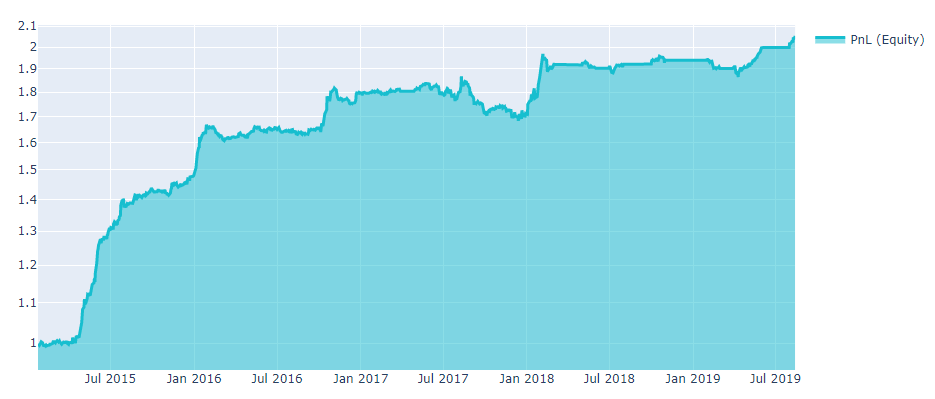Relative returns simply indicate how much the capital has changed. For the $$i^{th}$$ day we introduce the relative returns (rr) in unit fractions:

$\label{equity1} \text{rr}[i] = \frac{\text{equity}[i]}{\text{equity}[i-1]} - 1,$

Details

Sometimes it’s important to understand how equity (cumulative profit, PnL) is calculated. Say we allocate our capital in a proportion to the vector of weights for the $$i^{th}$$ day. Thus we buy shares at open price and receive the following positions:

$\label{position} \textbf{pos}[i] = \left( \textbf{weights}[i]\cdot\text{equity}[i] \right)/\textbf{open}[i],$

where bold variables stand for vectors in the space of shares; division is elementwise. For the next day, an algorithm will generate a new vector of weigths that will redistribute our capital into new positions. Redistribution of portfolio instruments leads to capital losses associated mainly with the broker’s commission and the slippage.

It is quite clear that the greater part of the capital we must redistribute, the more broker’s commission affects our profit. For real trading, slippage has a more significant impact on profit than the commission, so in our platform, we only consider slippage.

What is the slippage? We need a buyer/seller to sell/buy any shares. If there is no offer on the exchange, the order is opened at a new price. Thus, we buy the desired number of shares in parts, using offers to buy/sell a specific number of shares at a specific price. We calculate slippage according to the following formula:

$\label{slappage} \text{slippage}[i] = abs(\textbf{pos}[i] - \textbf{pos}[i-1])\cdot \textbf{ATR}(14) \cdot 0.05,$

where $$ \textbf{ATR}(14) $$ - is a market volatility indicator. The Average True Range ($$ \textbf{ATR}(N) $$) indicator is a moving average (MA) over N days of the true range (TR) values:

$\begin{gathered} \label{ATR} \textbf{TR}[i] = max( \textbf{high}[i] - \textbf{low}[i]; |\textbf{high}[i] - \textbf{close}[i-1]|; |\textbf{close}[i-1] - \textbf{low}[i]|), \end{gathered}$
$\label{ATR2} \textbf{ATR}(N) = MA(\textbf{TR},N).$

Now we can introduce the equity formula for the i day:

$\begin{split}\begin{gathered} \text{equity}[i] = \text{equity}[i - 1] + (\textbf{open}[i] - \textbf{close}[i-1]) \cdot \\ \textbf{pos}[i-1] + (\textbf{close}[i] - \textbf{open}[i]) \cdot \textbf{pos}[i] - \text{slippage}[i] \label{equity}\end{gathered}\end{split}$

## Algorithm quality¶

Once we have constructed an algorithm and plotted an equity on a historical data, we need to use a set of criteria to evaluate the performance.

### Sharpe Ratio¶

First, to estimate the profitability of the algorithm, we measure the Sharpe ratio (SR), the most important and popular metric. For our platform, we use the annualized SR and assume that there is ≈252 trading days on average per year. The annual SR formula for N days is presented below:

$\label{SR_final_first} \text{SR} = \frac{\sqrt[N]{[\prod\limits_{i=1}^{N} (rr_i + 1)]^{252}} - 1}{\sqrt{\frac{252}{N}\sum\limits_{i=1}^{N} (rr_{i} - \overline{rr}) }},$

where $$ rr_i $$ stands for the daily relative returns (of the i’th day), $$\overline{rr}$$ denotes the expected value.

The numerator is an average daily return. The book size changes with the size of equity, thus the numerator is a geometric mean.

The denominator is a standard deviation of the portfolio’s excess return. Another way to think about the denominator is that it means volatility.

Thus, the Sharpe ratio is the return per unit of risk (volatility). The greater the Sharpe ratio, the better (second figure). To submit a strategy successfully, the SR should be higher than 1 in the in-sample period.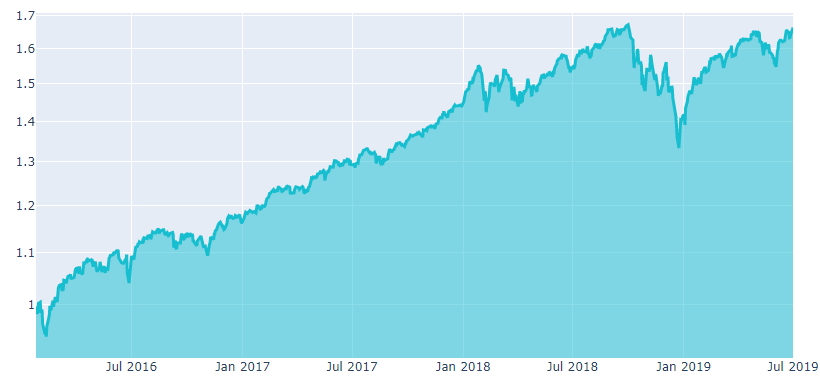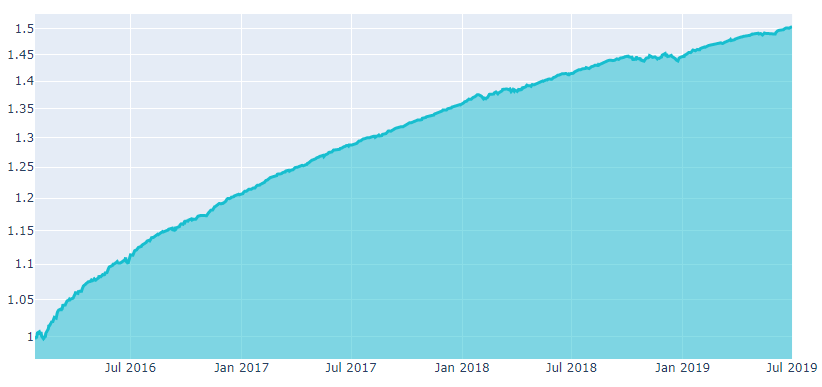Details

In 1994, William Sharpe defined the Sharpe ratio as:

$\label{SR_1} \text{SR} = \frac{E(R_p - R_f)}{\sigma_p},$

where $$ R_p $$ - return of the portfolio , $$ R_f $$ - risk-free rate, $$ E(R_p - R_f) $$ - s the expected value of the excess of the portfolio return over the benchmark return, $$ \sigma_p $$ - standard deviation of the portfolio’s excess return. We assume risk-free rate to be zero (alternative way to compute the Sharpe ratio is to set S&P 500 total return as a risk-free rate). For N trading days:

$\label{SR_2} \text{SR} = \frac{\text{average daily return}}{\text{daily volatility}} = \frac{\sqrt[N]{\prod\limits_{i=1}^{N} (rr_i + 1)} - 1}{\sqrt{\frac{1}{N}\sum\limits_{i=1}^{N} (rr_{i} - \overline{rr}) }},$

where $$ \overline{rr} $$ - denotes the expected value of relative returns. The book size changes with the size of equity, thus the numerator is a geometric mean. Now we introduce the SR, scaled on an arbitrary period $$ T $$:

$\label{SR_final} \text{SR} = \frac{\sqrt[N]{[\prod\limits_{i=1}^{N} (rr_i + 1)]^T} - 1}{\sqrt{\frac{T}{N}\sum\limits_{i=1}^{N} (rr_{i} - \overline{rr}) }}.$

For annual Sharpe ratio one can put T= 252 - trading days per year. We use the annual (T= 252) Sharpe ratio to estimate algorithms on our platform.

### Uniqueness¶

A good algorithm must minimize the overlap with well-known and already existing signals. Uniqueness can be defined in terms of the maximum correlation of the algorithm to the pool of the existing algorithms:

$r_{XY} = \frac{\text{cov}_{\textbf{X}\textbf{Y}}}{\sigma_{\textbf{X}} \sigma_{\textbf{Y}}}$

## In Sample and Out of Sample¶

Overfitting is easy. If one tries a significant amount of algorithm configurations, backtest can be fitted to any desired performance. Overfitting can be avoided by splitting the data into an In Sample and and an Out of Sample slices.

In sample

By “sample” we mean a data sample. An In-Sample (IS) set is the analogue of a training set in machine learning.

Out of sample

The out-of-sample (OS) set is the analogue of the testing set in machine learning. It is good practice to develop your algorithm on IS data and test it on OS unseen data.

Competition

The performace of a good algorithm OS does not degradate respect to the IS period.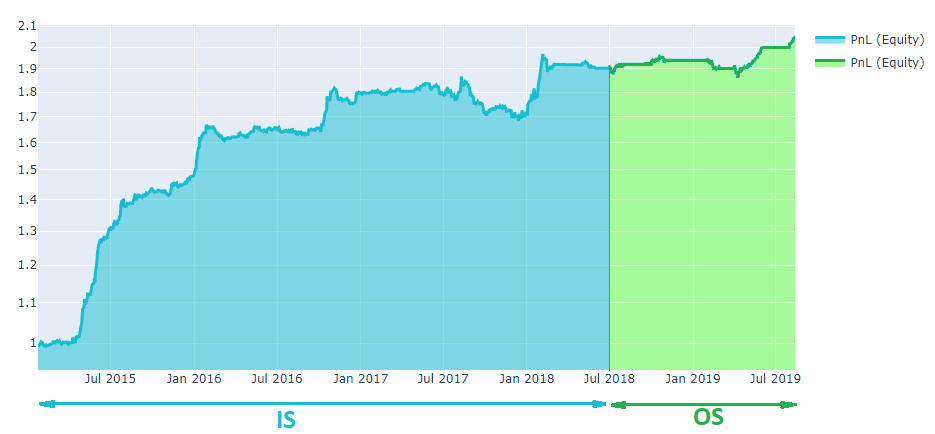## Improving the algorithm¶

### Neutralization¶

Let us analyse the stock performance of 500 large companies listed on stock exchanges in the United States, the so-called S&P500 index. As one can see, the market grows on average. S&P500 return vary widely from a few percent to over 20% in some years. Does it mean that simply opening a long positions is a good idea?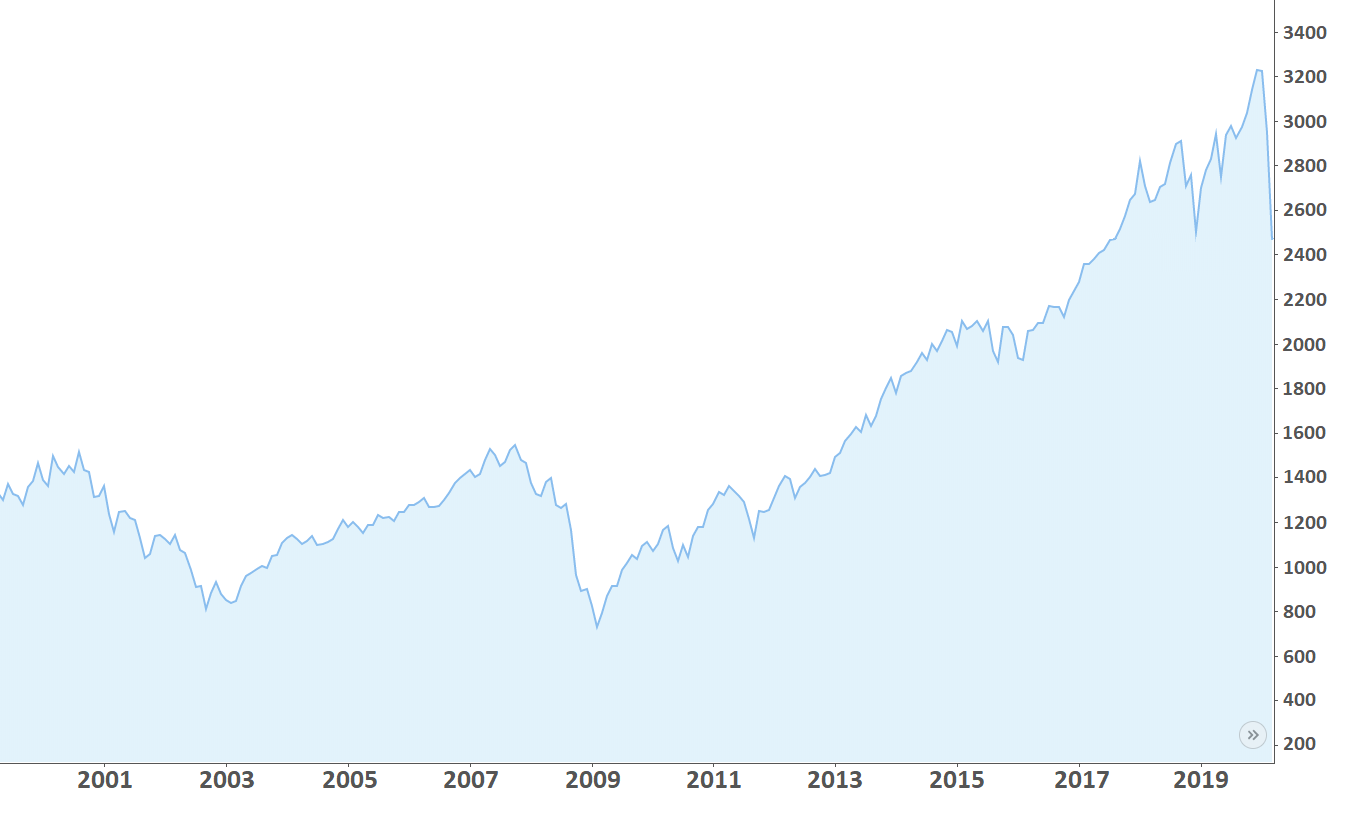Unfortunately, periodic financial crises occur:

• 1987, the Black Monday.

• 2000-2003, The Crash of the Dotcoms.

• 2007-2008. the Financial Crisis.

The consequences of crises are visible on the chart and appear as market drops of up to 30%. It is dangerous to think that crises are horror stories from the past.

We can exclude the market influence by balancing long/short positions for our algorithm. So, it will be a market-neutral algo. The neutralization could be done for the whole market or each industry (or smaller group). Mathematically, market neutralization is elementary.

Say, we a have a vector of weightsi for i day, given by the algorithm. In order to make the algorithm a market-neutral one, it is enough to apply the following equation for each day:

neutralized_weightsi = weightsi - mean(weightsi).

Now the mean of weights for each day is zero. It means that we neither invest money nor withdraw it from the market.